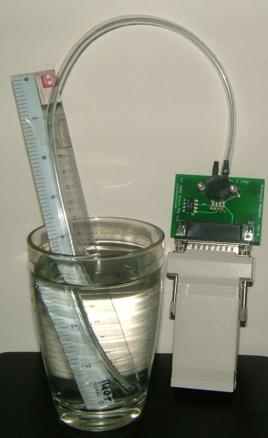The following information is for the EMANT300, EMANT380

# Measure Depth using Hydrostatic PressureThe pressure at a given depth of water is due the weight of the water acting on a unit area at that depth plus any pressure acting on the surface of the water.

If you measure the gauge pressure (pressure with respect to atmospheric pressure), the pressure due to water alone at a given depth is given

P = ρgh

where
P is the hydrostatic pressure
ρ is the water density
g is gravitational acceleration
h is the height of water above

One way to measure depth using hydrostatic pressure is to use the EMANT300 USB DAQ with the Pressure Application Adaptor and some aquarium airline tubing.

The application note Measure Pressure using silicon piezoresistive pressure sensor describes that the pressure in kPa with respect to the voltage output of the piezoresistive pressure sensor VO is given by

P = VO * 20000

Given that the conversion factor from kPa to cm H20 is 10.2, therefore the depth of water in cm is

hcm = 10.2 * P

Thus by adding this equation to the LabVIEW and .NET C# Pressure example program, you can now obtain the depth of water in cm.